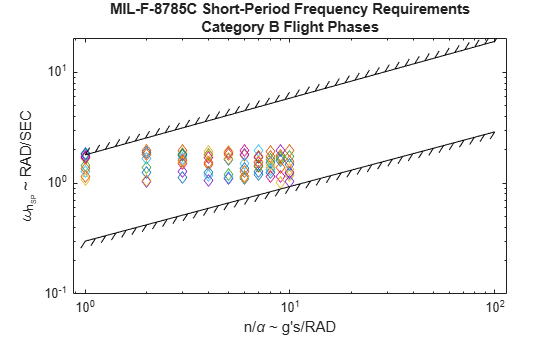# shortPeriodCategoryBPlot

Draw MIL-F-8785C short-period category B requirements plot

Since R2021b

## Syntax

``shortPeriodCategoryBPlot(nalpha,omega)``
``shortPeriodCategoryBPlot(nalpha,omega,LineSpec)``
``shortPeriodCategoryBPlot(nalpha1,omega1,LineSpec1,...nalphan,omegan,LineSpecn)``
``shortPeriodCategoryBPlot(___,Name,Value)``
``shortPeriodCategoryBPlot(ax,___)``
``[line,bline] = shortPeriodCategoryBPlot(___)``

## Description

### Basic Syntax and Line Specification

example

````shortPeriodCategoryBPlot(nalpha,omega)` plots vector `omega` versus vector `nalpha`. If `nalpha` or `omega` is a matrix, then the function plots the vector versus the rows or columns of the matrix, whichever are aligned. If `nalpha` is a scalar and `omega` is a vector, the function creates the disconnected line objects and plots them as discrete points vertically at `nalpha`. This function is based on the MATLAB® `plot` function. `shortPeriodCategoryBPlot(nalpha,omega,LineSpec)` plots short-period category A requirements specified by the line specification `LineSpec`. `shortPeriodCategoryBPlot(nalpha1,omega1,LineSpec1,...nalphan,omegan,LineSpecn)` combines the plots specified by the `nalpha`, `omega`, and `linespec`. It sets the line style, marker type, and color for each line. You can mix `nalpha`, `omega`, `LineSpec` triplets with `nalpha`, `omega` arguments, for example, `plot(nalpha1,omega1,nalpha2,omega2,LineSpec2,nalpha3,omega3)`.```

### Name-Value Arguments and Axes Specification

example

````shortPeriodCategoryBPlot(___,Name,Value)` plots an altitude envelope contour specified by one or more `Name,Value` arguments. Specify name-value arguments after all other input arguments. `shortPeriodCategoryBPlot(ax,___)` draws an altitude contour plot onto the axes `ax`. Specify arguments as previously listed after the `ax` argument. ```

### Return Line Objects

example

````[line,bline] = shortPeriodCategoryBPlot(___)` returns a vector of line objects `lineobjects` and a vector of `boundaryline` objects `boundary_lineobjects`. Use `lineobjects` and `boundary_lineobjects` to modify properties of a specific plot after it is created. Specify arguments as previously listed. ```

## Examples

collapse all

Plot the reference MIL-F-8785C short-period category B requirements.

`shortPeriodCategoryBPlot([])`Plot `nalpha` and `omega` data against the level 1 short-period category B requirements using diamond markers.

```nalpha = 1:10; omega = rand(10)+1; shortPeriodCategoryBPlot(nalpha,omega,"d","Level","1")```Plot the reference MIL-F-8785C short-period category B requirements. Return line objects and boundary line objects in h and b.

`[h,b] = shortPeriodCategoryBPlot([])````h = 0x1 empty Line array. ```
```b = 4x1 BoundaryLine array: BoundaryLine (Level 1) BoundaryLine (Level 1) BoundaryLine (Level 2) BoundaryLine (Level 2 & 3) ```

## Input Arguments

collapse all

Load factor per angle of attack n/α, specified as a scalar, vector, or matrix, in g's/radian.

Data Types: `double`

Short-period undamped natural frequency response ωnSP, specified as a scalar, vector, or matrix, in radians/second.

Data Types: `double`

Valid axes, specified as a scalar handle. By default, this function plots to the current axes, obtainable with the `gca` function.

Data Types: `double`

Line style, marker, and color, specified as a string scalar or character vector containing symbols. The symbols can appear in any order. You do not need to specify all three characteristics (line style, marker, and color). For example, if you omit the line style and specify the marker, then the plot shows only the marker and no line.

Example: `"--or"` is a red dashed line with circle markers.

Line StyleDescriptionResulting Line
`"-"`Solid line`"--"`Dashed line`":"`Dotted line`"-."`Dash-dotted lineMarkerDescriptionResulting Marker
`"o"`Circle`"+"`Plus sign`"*"`Asterisk`"."`Point`"x"`Cross`"_"`Horizontal line`"|"`Vertical line`"square"`Square`"diamond"`Diamond`"^"`Upward-pointing triangle`"v"`Downward-pointing triangle`">"`Right-pointing triangle`"<"`Left-pointing triangle`"pentagram"`Pentagram`"hexagram"`HexagramColor NameShort NameRGB TripletAppearance
`"red"``"r"``[1 0 0]``"green"``"g"``[0 1 0]``"blue"``"b"``[0 0 1]``"cyan"` `"c"``[0 1 1]``"magenta"``"m"``[1 0 1]``"yellow"``"y"``[1 1 0]``"black"``"k"``[0 0 0]``"white"``"w"``[1 1 1]`### Name-Value Arguments

Specify optional pairs of arguments as `Name1=Value1,...,NameN=ValueN`, where `Name` is the argument name and `Value` is the corresponding value. Name-value arguments must appear after other arguments, but the order of the pairs does not matter.

Before R2021a, use commas to separate each name and value, and enclose `Name` in quotes.

Example: `"Level","1"`

Note

These properties are only a subset. For a full list, see Line Properties.

Requirement level to plot, specified as:

• `"All"`

• `"1"`

• `"2"`

• `"3"`

Data Types: `double`

## Output Arguments

collapse all

One or more line objects, returned as a scalar or a vector. These are unique identifiers, which you can use to query and modify properties of a specific line. For a list of properties, see Line Properties.

One or more boundary line objects, returned as a scalar or a vector. These are unique identifiers, which you can use to query and modify properties of a specific chart line. For a list of properties, see Line Properties.

## Version History

Introduced in R2021b